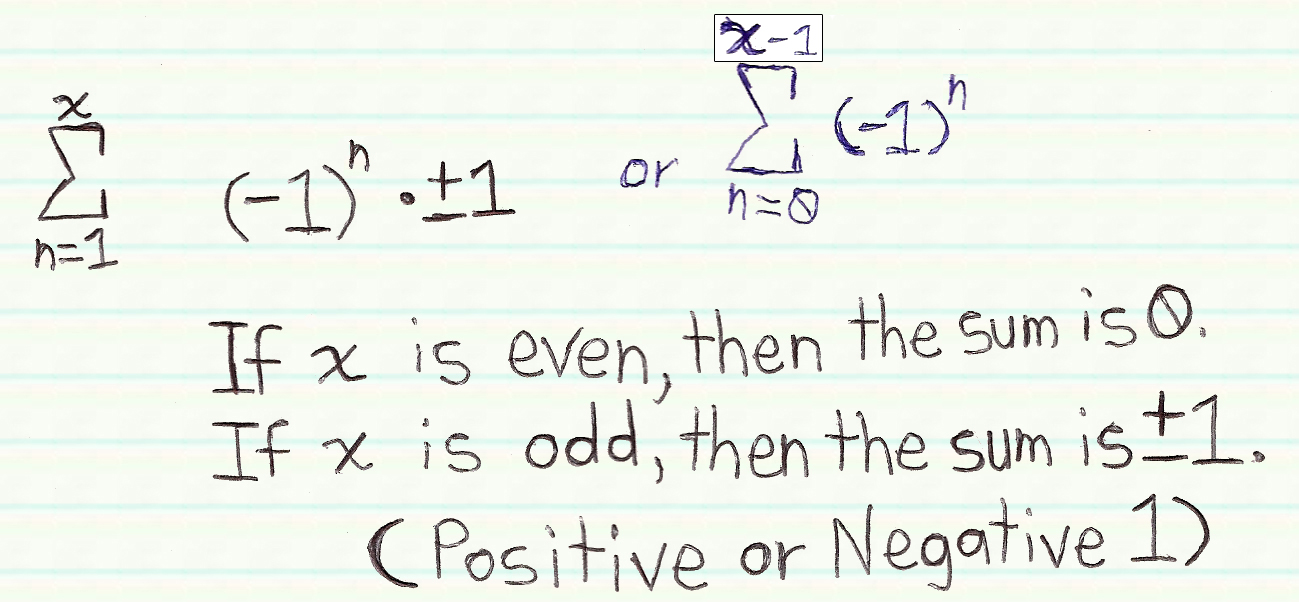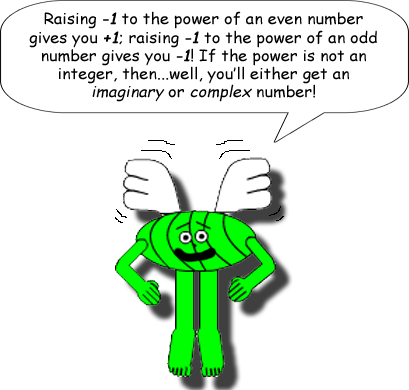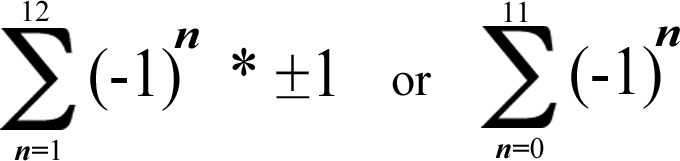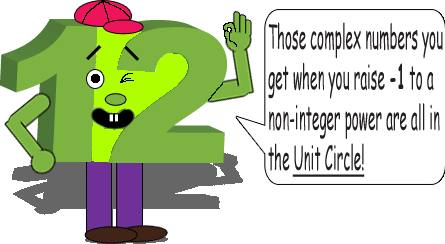# Super Summation 3

## This is another math trick that proves a number is even or odd. (Remember that only applies to integers.)## Examples:### (Yes, I'm using the asterisk as a multiplication symbol!)

-1 + 1 - 1 + 1 = 0 = 1 - 1 + 1 - 1

The summation equals 0, which proves that 4 is even.-1 + 1 - 1 + 1 - 1 = -1; 1 - 1 + 1 - 1 + 1 = +1

The summation equals ±1, which proves that 5 is odd.-1 + 1 - 1 + 1 - 1 + 1 - 1 + 1 - 1 + 1 - 1 + 1 = 0 = 1 - 1 + 1 - 1 + 1 - 1 + 1 - 1 + 1 - 1 + 1 - 1

The summation equals 0, which proves that 12 is even.This number person character is Mr. Twelve Teeneet.